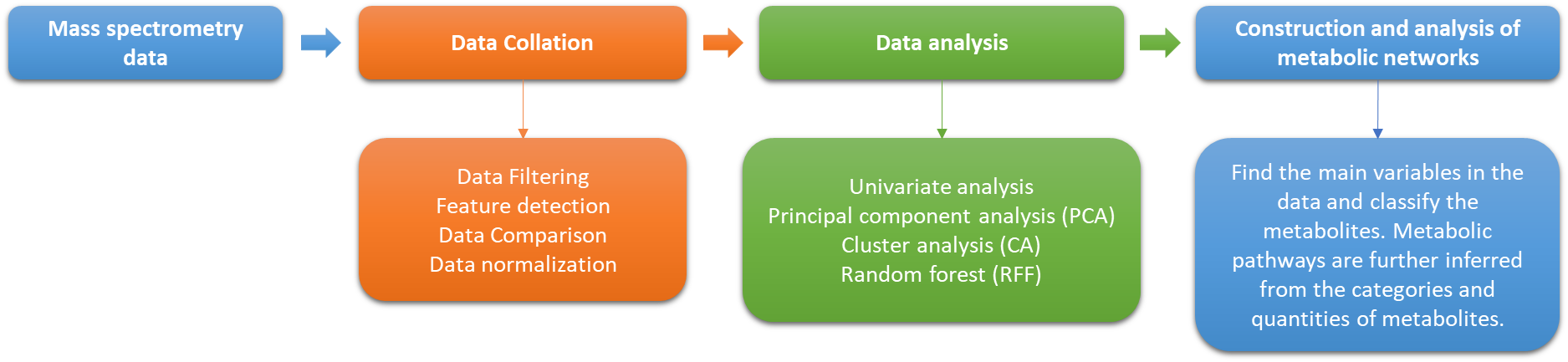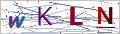Creative Proteomics Metabolomics

# Metabolomics Data Analysis

## Metabolomics Data Analysis

Online Inquiry

Metabolomic analysis of biological samples by high-throughput instruments such as GC-MS, LC-MS, etc. results in large and complex datasets and therefore requires extensive algorithms and workflows to process and analyze the information obtained. Creative Proteomics provides metabolomic bioinformatics analysis services for data visualization.

We offer metabolomic analysis services, including basic bioinformatics analysis of the acquired data, as well as custom advanced bioinformatics analysis. Alternatively, you can provide the acquired mass spectrometry data directly and we will customize the bioinformatics analysis service for you.1. Q: How to do further screening for differential metabolites?

A: Screening for differential metabolites is generally performed using a combination of thresholds such as P-value < 0.05 vip=" ">1.

P-values are derived from unidimensional statistical analysis (e.g., T-test). VIP values are derived from multivariate statistical analysis (e.g., OPLS-DA) characterizing the value of the contribution of that variable to the difference between the two groups.

There is also a practice of using P-values <0.05 logfc="">1 or logFC<-1) [FC=Fold change] for differential screening, but both P-values and FC values are sourced from univariate statistical analysis.

To do further screening on this basis, there are several approaches.

(1) P-value < 0.05, ranking the VIP values (the larger the VIP value, the more significant the differential metabolite).

(2) VIP>1, to rank the P values (the smaller the P value, the more significant the differential metabolite).

(3) Within the range of P values <0.05 vip=" ">1, do ranking on logFC values (the larger the logFC greater than 1, the more significant; the smaller the logFC less than -1, the more significant).

Restricted to stricter base screening conditions, e.g., p-value <0.01& vip=" ">2.

2. Q: What is the volcano chart and what is it for?

A: The volcano map mainly shows the information of two dimensions, P-value and Fold-Change. All these information is closely related to the screening of differential metabolites, so it shows the distribution profile of differential metabolites in all substances.

3. Q: What is a Venn diagram? What is the use of it?

A: A Venn diagram is used to show all possible logical relationships between a finite set of different.

4. Q: What do R2 and Q2 mean respectively?

A: R2X (for PCA) or R2Y (for PLS-DA) indicates the proportion of the data variance or variance that can be explained by the current model, indicating the goodness of fit (the fit). Q2 indicates the proportion of the data variance that can be predicted by the current model, i.e., the prediction rate, indicating the predictive power of the current model.

5. Q: What is the difference between PLS-DA and OPLS-DA models?

A: OPLS-DA has an additional positive exchange calculation than PLS-DA, which filters out signals that are not relevant to the model classification. For example, if the difference between groups is relatively small and the difference within groups is relatively large, the PLS-DA VIP is used to screen out what may be the within-group difference variable, which is easily misleading. OPLS-DA is an upgraded version of PLS-DA.

6. Q: Permutation test judgment criteria?

A: The replacement test is the randomization test or re-randomization test. The usual criteria are R2<0.3 and Q2<0.05, but sometimes the sample biology is too small to meet the requirements, so only the slope of the regression line needs to be positive.

7. Q: Why is the comparative analysis only two by two?

A: Because in the search for differentials it is determined whether the substance is a differential based on the difference in content. The amount of a substance in one group rises/declines relative to the other group, while the amount of change relative to the other two groups cannot be calculated at the same time.

8. Q: Can the sample sizes be different for the two comparison groups?

A: Yes, only the minimum number of biological replicates in each group is required to be met.

9. Q: Can data from multiple platforms be integrated into one piece for PCA?

A: Yes.

For Research Use Only. Not for use in diagnostic procedures.

## Connect with Creative ProteomicsContact Us